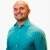# Solving a literal equation by solving for y with a fractionThis lesson will look at an example problem involving literal equations. This lesson will give an explanation of solving a literal equation by solving for y with a fraction with a step by step look to... This lesson will look at an example problem involving literal equations. This lesson will give an explanation of solving a literal equation by solving for y with a fraction with a step by step look to better explain the concept.
More... Collapse
1 Views

## Comments (0)

Please log in to post comments.